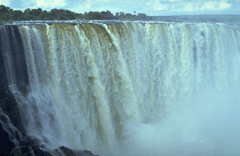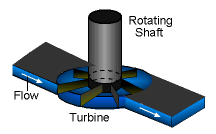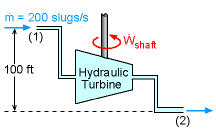Ch 4. Fundamental Laws (Integral Anal.) Multimedia Engineering Fluids ConservationMass LinearMomentum Moment ofMomentum ConservationEnergy
 Chapter 1. Basics 2. Fluid Statics 3. Kinematics 4. Laws (Integral) 5. Laws (Diff.) 6. Modeling/Similitude 7. Inviscid 8. Viscous 9. External Flow 10. Open-Channel Appendix Basic Math Units Basic Equations Water/Air Tables Sections Search eBooks Dynamics Fluids Math Mechanics Statics Thermodynamics Author(s): Chean Chin Ngo Kurt Gramoll ©Kurt GramollFLUID MECHANICS - CASE STUDY IntroductionElectricity Generated using Water PowerTurbineProblem Description Hydroelectric power is the largest source of renewable energy produced in the United States. As water flows through turbines, mechanical energy in terms of shaft work is produced, and electricity is generated by converting this mechanical energy through generators. Consider a turbine with the inlet and outlet conditions as shown in the figure. The water flows at a rate of 200 slugs/s. The pressure drop is 50 psi across the 100 ft elevation difference between the inlet and outlet. The inlet and outlet pipe radii are 2 ft and 3 ft, respectively. How much power will the hydraulic turbine produce? Questions Determine the rate of work done (power) produced by the turbine. Approach Assume the flow is steady. Assume the hydraulic turbine has no power loss (i.e., adiabatic and no change in the internal energy). The density of the water is 1.94 slugs/ft3.

Practice Homework and Test problems now available in the 'Eng Fluids' mobile app
Includes over 250 problems with complete detailed solutions.
Available now at the Google Play Store and Apple App Store.